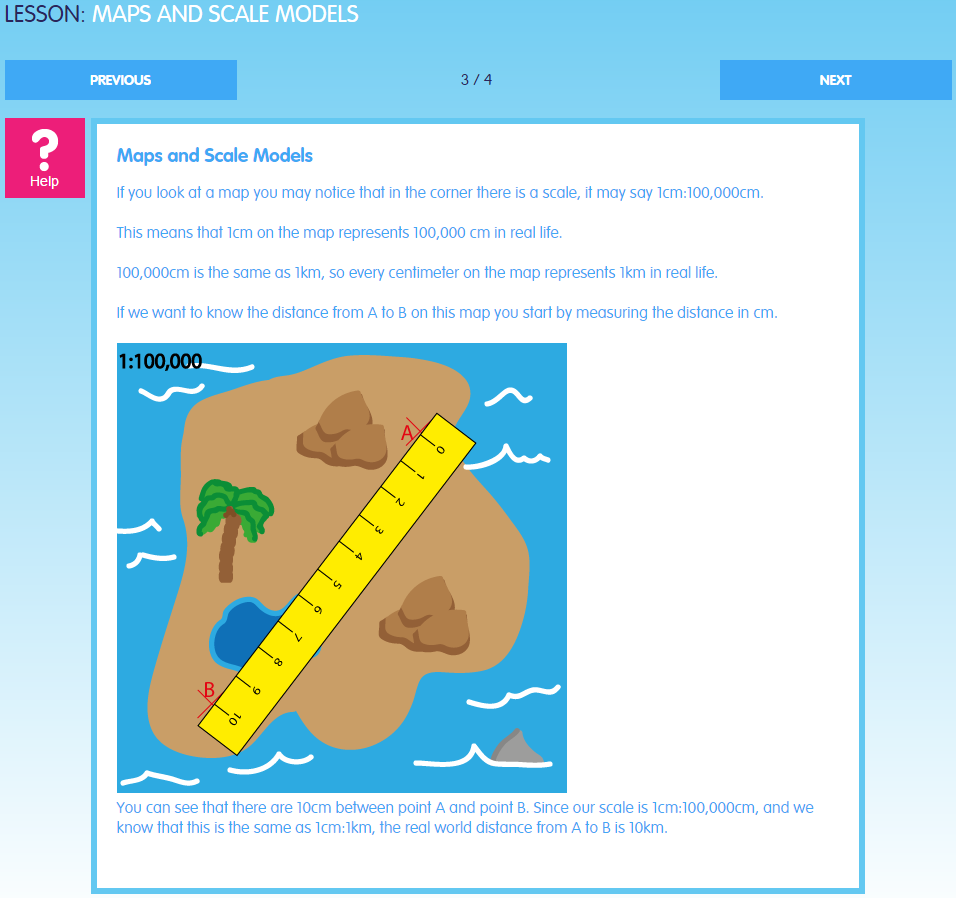## Subjects and Courses

Our range of Mathematics content enables any learner to progress, regardless of ability. Learners follow a personalised pathway, structured to enhance their knowledge and understanding of Mathematics.

We have mapped the content to the Mathematics National Curriculum for Key Stages 1 - 4 and to the GCSE Mathematics Specifications to incorporate the following topics:

• Algebra
• Geometry: Position and Direction
• Geometry: Properties of Shapes
• Geometry and Measures
• Measurement
• Multiplication and Division
• Number
• Number: Addition, Subtraction, Multiplication and Division
• Number: Fractions (Including Decimals and Percentages)
• Number and Place Value
• Probability
• Ratio, Proportion and Rates of Change
• StatisticsThese Mathematics topics can be further broken down to show the extensive range of lessons available in each area: